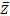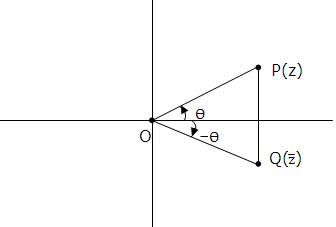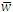×#### Thank you for registering.

One of our academic counsellors will contact you within 1 working day.

Click to Chat

1800-1023-196

+91-120-4616500

CART 0

• 0

MY CART (5)

Use Coupon: CART20 and get 20% off on all online Study Material

ITEM
DETAILS
MRP
DISCOUNT
FINAL PRICE
Total Price: Rs.

There are no items in this cart.
Continue Shopping```Conjugate of a Complex NumbersConjugate of a complex number z = a + ib is denoted and defined by= a-ib.

In a complex number if we replace i by -i, we get conjugate of the complex number.is the mirror image of z about real axis on Argand's Plane.

Geometrical representation of conjugate of complex number -

|z| = ||

arg () = - arg (z)

General value of arg ()= 2nΠ P.V. arg(z)Properties:

(i) If z = x+y, then x= z+/2, y = z+/2

(ii) z == z is purely real

(iii) z += 0 = j is purely imaginary

(iv) ||2 = z(v)= z(ix) Imaginary roots of polynomial equations with real coefficient occur is conjugate pairs.

(x) If w=f(z), then= f()

To read more, Buy study materials of Complex Numbers comprising study notes, revision notes, video lectures, previous year solved questions etc. Also browse for more study materials on Mathematics here.
```### Course Features

• 731 Video Lectures
• Revision Notes
• Previous Year Papers
• Mind Map
• Study Planner
• NCERT Solutions
• Discussion Forum
• Test paper with Video Solution